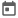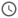# Verilog System Functions##### 23 Sep 2021##### 4 mins

There are various helper functions defined in the language itself, which makes specific tasks easy. These functions are known as system functions. In Verilog, various system functions help perform critical tasks like console log some information, read data from a file, write data to file, terminate the simulation, and many others. According to their operation, these functions have been categorized into different sections to understand the various system functions better. This categorization is not a Verilog standard but used to make learning easier.

### Console Log functions

As the name suggests, these functions help to print log information in the console. A log is any information printed by the code while the simulation is running. Displaying logs are very beneficial to debug the code. Verilog provides three different functions for this operation.

#### \$display

This function is the most commonly used function to print logs in the console. This function prints the information passed as the argument in the console immediately, i.e., in the active region.

#### \$strobe

This function is the same as that of the display statement. The only difference is that it will not print the statement immediately. However, it will print the information in the postponed region. Thus the value of variable printed using \$strobe will always be free of any race condition.

#### \$monitor

This function, like the strobe statement, prints the information in the postponed region. However, this function behaves differently than a strobe function. \$monitor will automatically print the log if any of the variables which are logged change. Thus, once defined, it will print the log every time the variable changes. Do not worry this concept will be clear once we see an example

#### Format Specifiers

These specifiers are used in the string that is passed as an argument to the log functions. For a different types of variables, there are different specifiers. Specifiers can be considered a placeholder for a variable, which will be replaced with the variable’s value during runtime. For example, `\$display(“a = %b”, a);` in this display statement, `%b` is the format specifier and will be replaced by the value of the a in binary base.

Different format specifiers available in Verilog are as follows.

SpecifierDescription
%b, %BDisplays the variable in binary base
%h, %HDisplays the variable in hexadecimal base
%d, %DDisplays the variable in decimal base
%o, %ODisplays the variable in octal base
%s, %SDisplays variable as a string. This modifier will convert ASCII to characters and display it.
%t, %TDisplay the output as time. Generally used with \$time or \$realtime system functions
%p, %PThis modifier is used to display arrays. In this case, the values are by default shown in decimal base. To print in other base, \$displayh, \$displayo or \$displayb can be used
%f, %FTo print variables of real datatype.
%e, %EDisplays variable of real data type in exponential format.
%m, %MDisplays hierarchical name. not used generally, but can help debug.

Format specifier may leave a blank space before printing the value. The blank space can be removed by adding `0` in between % and specifier code, for example, `%0d`, `%0h`, etc. Also, in real value, the number of decimal places can be controlled using a format specifier. For example, `%0.00f` will print two decimal places.

#### Escape Characters

While writing string for logging, sometimes special characters like tab, newline, “, needs to be used. These characters cannot be directly used, and Verilog provides some escape characters which can be used instead.

Escape characterDescription
\nNewline
\tTab
\”To insert `“` in string
\To print \ in string
%%To print %

#### Example 1

``````module display_demo;
reg [3:0] a;
real b;
reg [1:0] arr [2:0];
integer i;

initial begin
a = 4'b1011;
b = 2.52;
for (i=0; i<3; i=i+1) begin
arr[i] = 2-i;
end

// Display with different format modifier
\$display("a = %b", a);
\$display("a = %h", a);
\$display("a = %o", a);
\$display("a = %d", a);
\$display("b = %f", b);
\$display("b = %e", b);
\$display("arr = %p", arr);
\$displayb("arr = %p", arr);
\$displayh("arr = %p", arr);
end
endmodule``````
##### Output
``````# a = 1011
# a = b
# a = 13
# a = 11
# b = 2.520000
# b = 2.520000e+000
# arr = '{0, 1, 2}
# arr = '{00, 01, 10}
# arr = '{0, 1, 2}``````

#### Example 2

In this example, we can see that the `\$monitor` prints the log every time the variable changes. Also, we can see that the `\$display` is printed first because it executes in the active region. `b` is initialised with a non-blocking assignment. Thus the `\$display` prints the previous value of `b`, and in `\$strobe`, the new value is seen.

``````module log_demo;
reg [7:0] a, b;
initial begin
\$monitor("MONITOR: a = %0d", a);
end
initial begin
a = 8'd8;
b = 8'd3;
#10;
a = 8'd15;
b <= 8'd35;
\$display("DISPLAY: b = %0d", b);
\$strobe("STROBE: b = %0d", b);
end
endmodule``````
##### Output
``````# MONITOR: a = 8
# DISPLAY: b = 3
# MONITOR: a = 15
# STROBE: b = 35``````

### Randomisation Functions

As the name suggests, these functions generate a random number. They are mainly used in testbench to create random test vectors.

#### \$random

This function generates a random 32bit signed number.

#### \$urandom

This function generates a 32bit random unsigned number.

#### \$urandom_range

This function also generates a 32-bit random unsigned number but inside the range mentioned.

#### Example

``````module random_demo;
reg [31:0] a, b;
integer c;

initial begin
repeat(10) begin
a = \$urandom();
b = \$urandom_range(1, 8);
c = \$random();
\$display("a = %0d,\t\t\tb = %0d,\t\t\tc = %0d", a, b, c);
end
end
endmodule``````
##### Output
``````# a = 3948585912,			b = 7,			c = 303379748
# a = 2634922342,			b = 7,			c = -1064739199
# a = 1359874246,			b = 7,			c = -2071669239
# a = 1003556722,			b = 7,			c = -1309649309
# a = 4197925991,			b = 3,			c = 112818957
# a = 1190730858,			b = 5,			c = 1189058957
# a = 1628895855,			b = 7,			c = -1295874971
# a = 2618897973,			b = 5,			c = -1992863214
# a = 345981102,			b = 6,			c = 15983361
# a = 852277211,			b = 6,			c = 114806029``````

### Time Functions

These functions return present simulation time.

#### \$time

This function returns the time in terms of the time unit mentioned. The decimal part of the time step is rounded up.

#### \$realtime

This function returns the time in terms of the time precision mentioned.

#### Example

In this example, it can be seen that when we have a delay of 1.4, `\$time` gives the output in terms of the time unit that is 10ns. In comparison, the `\$realtime` gives the output in terms of time precision, i.e., 1ns.

```````timescale 10ns/1ns
module time_demo;
initial begin
#1
\$display("Time is %0t", \$time);
\$display("RealTime is %0t", \$realtime);

#1.4
\$display("Time is %0t", \$time);
\$display("RealTime is %0t", \$realtime);
end
endmodule``````
##### Output
``````# Time is 10
# RealTime is 10
# Time is 20
# RealTime is 24``````

### Miscellaneous

\$stop – Stops the simulation

\$finish – Stops the simulation and closes the simulator.